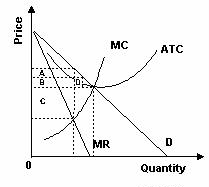### CFA Practice Question

There are 539 practice questions for this study session.

### CFA Practice Question

Refer to the graph below. Which rectangle represents monopolists' profit?A. A
B. A + B + C
C. C + D
Explanation: Output is determined where marginal revenue equals marginal cost. Profit is determined by the rectangle created by the relevant price and average cost at that output.